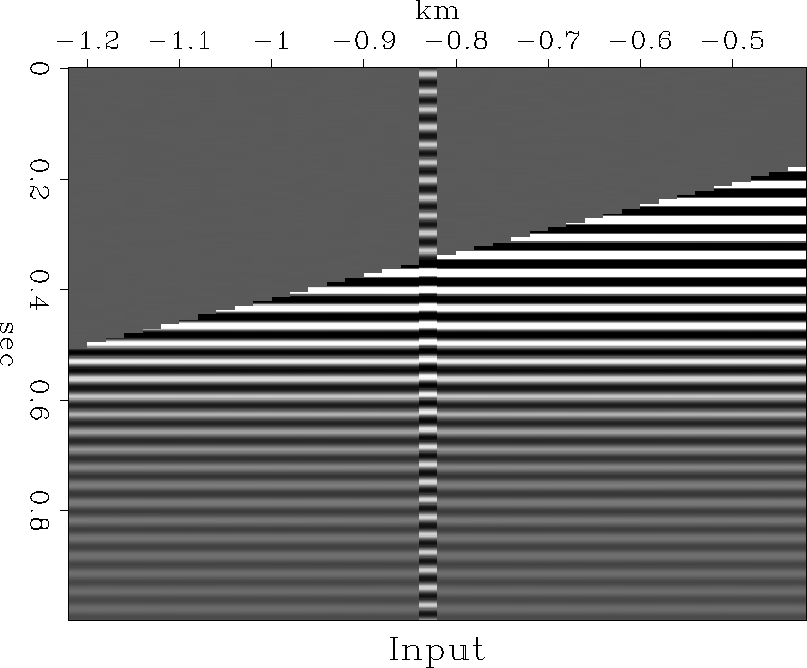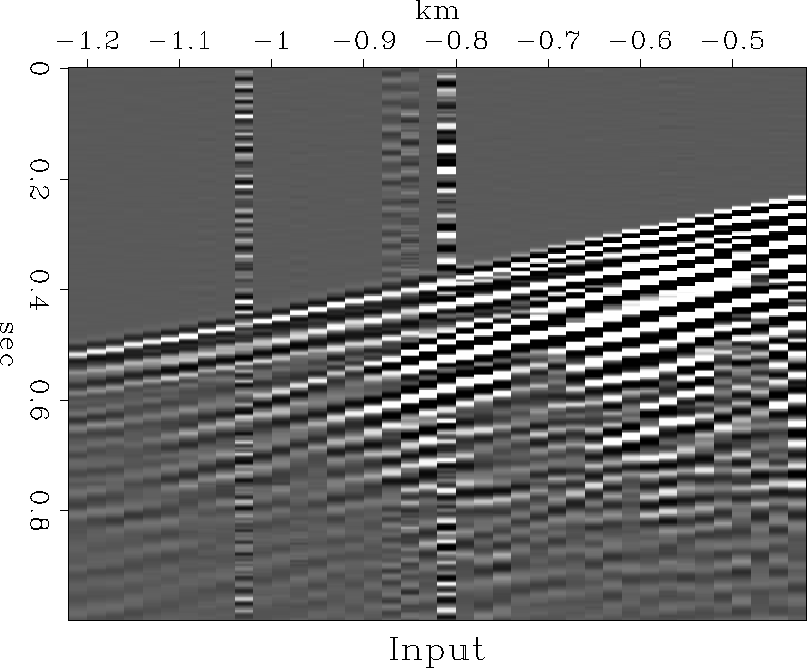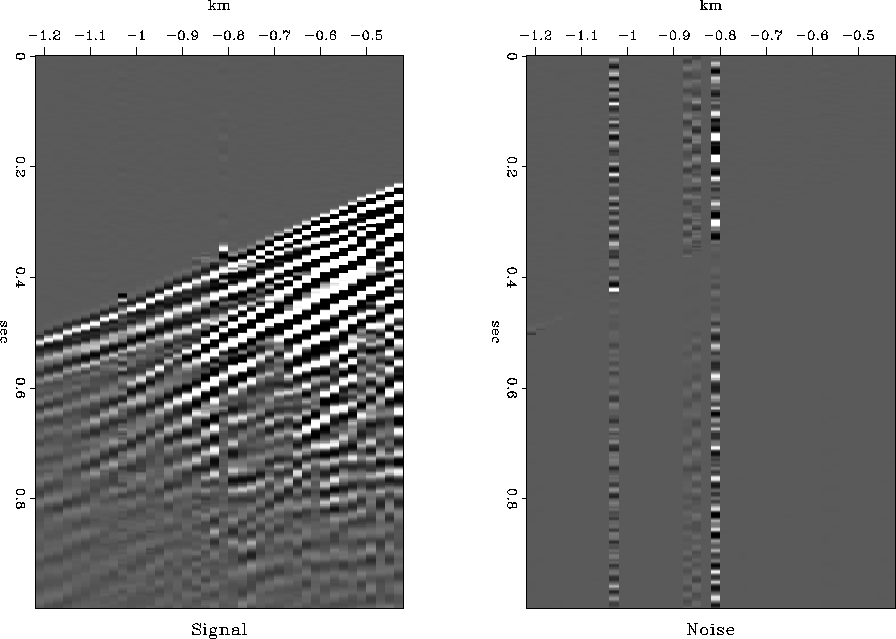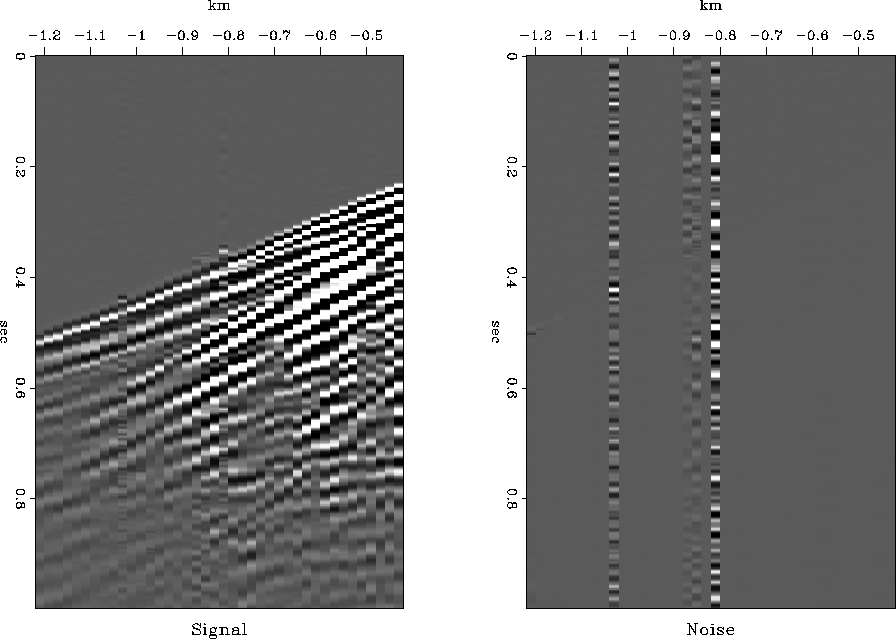# Short Note Enhanced prestack noise removal

Ray Abma

ray@sep.stanford.edu

In a previous paper, Abma and Claerbout, presented a technique for separating signal and noise. This technique was fairly expensive since it used an inversion routine that required many iterations to get a reasonable result. Generally the process worked well, but occasionally the traces that contained high amplitude noise showed weak signal on the output. The cause of both the high cost and erratic results can be attributed to the difficulty the inversion routine had in predicting noise. With spiky noise, the inversion was unlikely to reconstruct the noise accurately, since noise is generally broadband and will not be easily reconstructed from the response of a filter that has a bandlimited response.

In this paper, I improve the results of the inversion by initializing the noise estimate with the result of a lateral prediction filter. This initialization is similar to that used in Abma except that the process is applied to prestack data and the inversion is unchanged from Abma and Claerbout. This initialization improves the results and reduces the cost of the process.

I show comparisons of the results of using the inversion with the initial noise estimate being zero as in Abma and Claerbout and then with the initial noise estimate being the result of a lateral prediction filter on both synthetic and real data.

INITIALIZING THE INVERSION

In Abma and Claerbout, a system of regressions for signal and noise separation was solved using an initial value of noise n that was zero. This initial value of n requires the inversion to fully calculate the noise. The approach suggested in this note is to use an estimate of the noise n that is Fs d, the result of the signal annihilation filter applied to the data. When the signal annihilation filter Fs is applied to the data d, all the signal should be removed. The noise, which is not predicted by Fs, will remain, although the noise is modified somewhat by Fs.

This approach is similar to that of Abma in the post-stack case, where the result of prediction filtering is used to estimate a preliminary guess of the noise before the inversion. The difference between the two methods is that in the post-stack case the estimate of the noise n, Fs d, is also used as a stabilizer for the inversion. In the prestack case, this stabilization appears to be unnecessary.

Another difference between the method applied here and the post-stack method is that the signal annihilation filter Fs is recalculated iteratively. To achieve a similar effect in the prestack case, I intend to reduce the effect of the noise on the signal annihilation filter by removing the worst of the noise with prediction error amplitude criteria. This technique will be covered in a later work.

EXAMPLES

Figure sinefile5 shows a synthetic shot gather with a trace containing noise of the same frequency as the signal. This case presents a problem that normally is difficult to solve, since the separation of signal and noise cannot be done by simple frequency separation. Figure sf2wbq.c shows the result of using the inversion from Abma and Claerbout with an initial value of the noise being zero. Notice that the signal is corrupted well up into the first arrival area and the noise display shows that the noise, which should have constant amplitude in time, is weakened in the first arrival area. Figure sf2wcq.c shows the result of using the same inversion, but with an initial value of the noise being Fs d, the result of the signal annihilation filter applied to the data. The result shown in Figure sf2wcq.c is much improved over that shown in Figure sf2wbq.c, which shows that initializing the noise with Fs d improves the solution. Also, the number of iterations was reduced by a factor of 10 in the case where the noise was initialized with Fs d. While the separation is not yet perfect, the noise display shows that the extracted noise now has a more constant amplitude in time, as it should.sinefile5
Figure 1
A simple synthetic showing noise and signal with the same frequency.sf2wbq.c
Figure 2
A simple example of separation of signal and noise using sine waves of the same frequency. The inversion was used with the noise initialized to zero. The plot on the left is the calculated signal, and the plot on the right shows the noise that remained.sf2wcq.c
Figure 3
A simple example of separation of signal and noise using sine waves of the same frequency. The inversion was used with the noise initialized to Fs d. The plot on the left is the calculated signal, and the plot on the right shows the noise that remained.

Figure original shows another input to the noise separation: a real dataset with noisy traces. Figure sf2wbq.a shows the result of using the inversion on this shot gather with an initial value of the noise being zero. Figure sf2wcq.a shows the result of using the same inversion, but with an initial value of the noise being Fs d. Once again, the results are improved and the cost is reduced.original
Figure 4
The input shot gather with some noise.sf2wbq.a
Figure 5
A simple example of separation of signal and noise from the shot gather in the previous figure. The inversion was used with the noise initialized to zero. The plot on the left is the calculated signal, and the plot on the right shows the noise that remained.sf2wcq.a
Figure 6
A simple example of separation of signal and noise on a shot gather. The inversion was used with the noise initialized to Fs d. The plot on the left is the calculated signal, and the plot on the right shows the noise that remained.

CONCLUSIONS

The results of a previous method of separating signal and noise was improved by initializing the noise to an estimate of the noise derived from lateral prediction filtering before entering the inversion routine. In addition to the improved results, the cost of the method is greatly reduced. While this method is not yet perfected, it may be possible to get other improvements by zeroing high amplitude noise before the least-squares inversion, which would then predict the zeroed data simultaneously with separation of signal and noise.

[mybib]